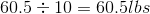# AP Statistics : How to find the mean of the sum of independent random variables

## Example Questions

### Example Question #1 : How To Find The Mean Of The Sum Of Independent Random Variables

Ifis a random variable with a mean ofand standard deviation of, what is the mean and standard deviation of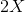?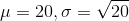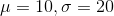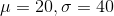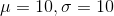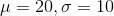Explanation:

Remember how the mean and standard deviation of a random variable are affected when it is multiplied by a constant.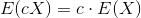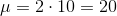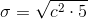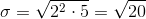### Example Question #2 : How To Find The Mean Of The Sum Of Independent Random Variables

If you have ten independent random variables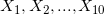, normally distributed with meanand variance, what is the distribution of the average of the random variables,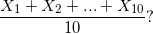Normal distribution with with meanand variance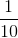.

Chi-square distribution withdegrees of freedom.

Normal distribution with meanand variance.

Normal distribution with meanand variance.

Normal distribution with with meanand variance.

Explanation:

Any linear combination of independent random variables is also normally distributed with the mean and variance depending on the weights on the random variables. The mean is additive in the sense that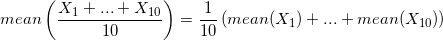Each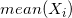is, so the sum is equal to zero.

This means the sum of the average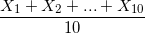is.

The variance satisfies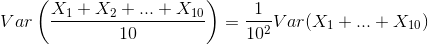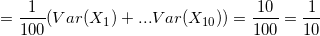because of independence.

This means that the average is normally distributed with meanand variance.

### Example Question #3 : How To Find The Mean Of The Sum Of Independent Random Variables

Suppose you have three independent normally distributed random variables,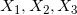, such that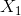has meanand variance,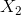has meanand variance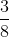,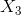has meanand variance.

What is the probability that the sum,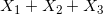, is less than?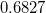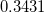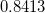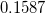Explanation:

There is a relatively simple way of doing this problem. The sum of any set of independent normal random variables is also distributed normally. Sohas a normal distribution. Now we can compute the mean and variance. The mean is additive: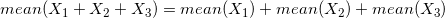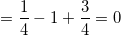Variance is also additive in some sense, when the random variables are independent: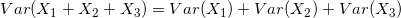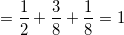Thus,is normally distributed with meanand variance.

This sum is a standard normal distribution.

The chance that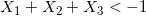is thus, if we use a normal table.

### Example Question #4 : How To Find The Mean Of The Sum Of Independent Random Variables

An experiment is conducted on the watermelons that were grown on a small farm.  They want to compare the average weight of the melons grown this year to the average weight of last year's melons.  Find the mean of this year's watermelons using the following weights: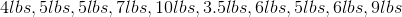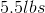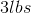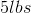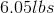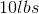To find the mean you sum up all of your values then divide by the total amount of values.  The total sum of the weights is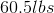and there are 10 melons.